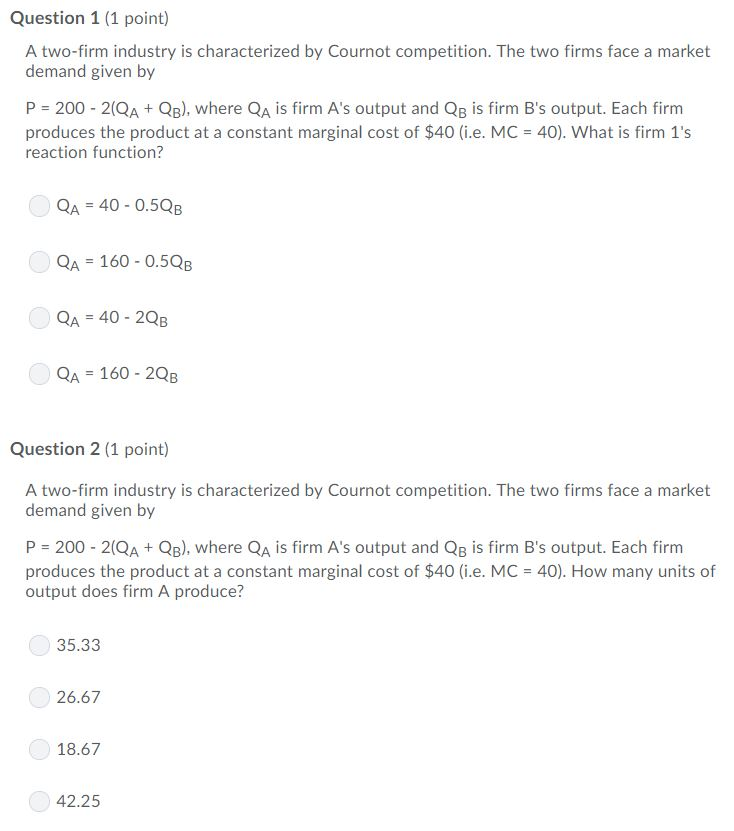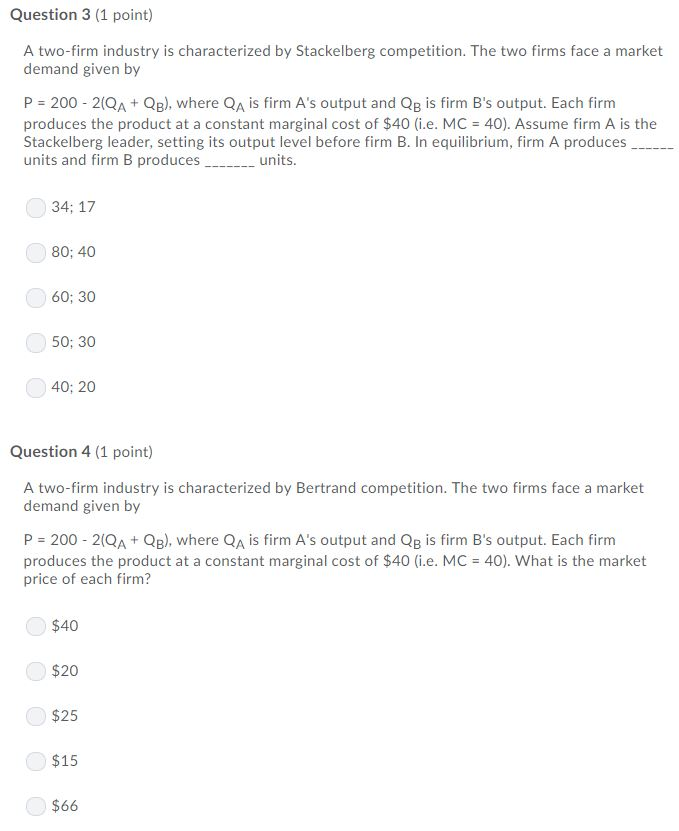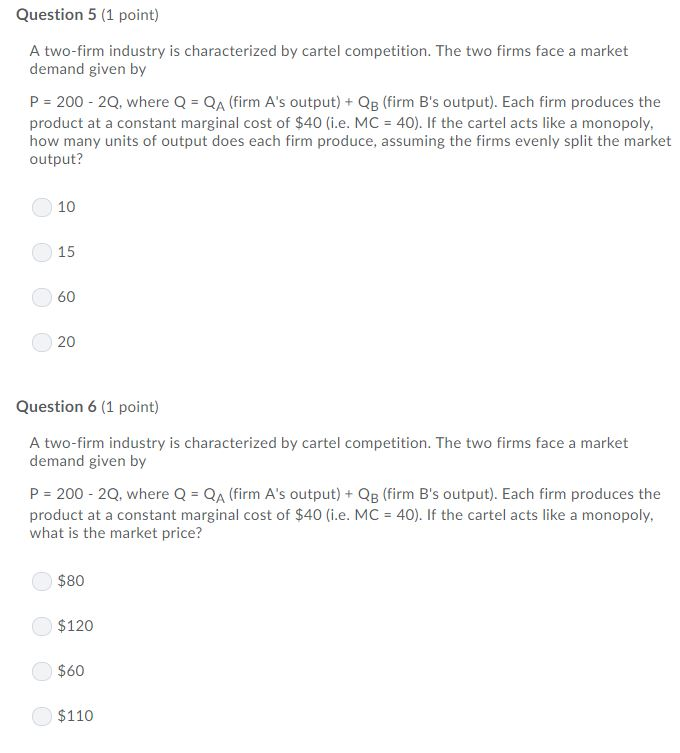### Create an Account

Home / Questions / Question 1 (1 point) A two-firm industry is characterized by Cournot competition. The two firms face

# Question 1 (1 point) A two-firm industry is characterized by Cournot competition. The two firms faceQuestion 1 (1 point) A two-firm industry is characterized by Cournot competition. The two firms face a market demand given by P 200 2(QA+QB), where QA is firm A&#39;s output and QB is firm B&#39;s output. Each firm produces the product at a constant marginal cost of \$40 (i.e. MC 40). What is firm 1&#39;s reaction function? QA= 40-0.5QB QA 160 0.5QB QA = 40 2QB QA 160 2QB Question 2 (1 point) A two-firm industry is characterized by Cournot competition. The two firms face a market demand given by P 200 2(QA+QB), where QA is firm A&#39;s output and QB is firm B&#39;s output. Each firm produces the product at a constant marginal cost of \$40 (i.e. MC 40). How many units of output does firm A produce? 35.33 26.67 18.67 42.25 Question 3 (1 point) A two-firm industry is characterized by Stackelberg competition. The two firms face a market demand given by P 200 2(QA+ QB), where QA is firm A&#39;s output and QB is firm B&#39;s output. Each firm produces the product at a constant marginal cost of \$40 (i.e. MC 40). Assume firm A is the Stackelberg leader, setting its output level before firm B. In equilibrium, firm A produces units and firm B produces - units. 34; 17 80; 40 60; 30 50; 30 40; 20 Question 4 (1 point) A two-firm industry is characterized by Bertrand competition. The two firms face a market demand given by P 200 2(QA+ QB), where QA is firm A&#39;s output and QB is firm B&#39;s output. Each firm produces the product at a constant marginal cost of \$40 (i.e. MC 40). What is the market price of each firm? \$40 \$20 \$25 \$15 \$66 Question 5 (1 point) A two-firm industry is characterized by cartel competition. The two firms face a market demand given by P 200 2Q, where Q QA (firm A&#39;s output) + QB (firm B&#39;s output). Each firm produces the product at a constant marginal cost of \$40 (i.e. MC 40). If the cartel acts like a monopoly, how many units of output does each firm produce, assuming the firms evenly split the market output? 10 15 60 20 Question 6 (1 point) A two-firm industry is characterized by cartel competition. The two firms face a market demand given by P 200 2Q, where Q QA (firm A&#39;s output)+ Qp (firm B&#39;s output). Each firm produces the product at a constant marginal cost of \$40 (i.e. MC 40). If the cartel acts like a monopoly, what is the market price? \$80 \$120 \$60 \$110

Apr 03 2020 View more View LessSubscribe To Get Solution